# Risk Analysis (Monte Carlo Simulation)

## Perform Monte Carlo Risk Analysis with any assumptions you choose versus any measure, such as Rate of Return (IRR or MIRR), Net Present Value (NPV), etc. Risk Analysis allows you to investigate how these measures vary with a change in assumptions like Holding Period, Cap Rate at Sale, Renewal Probability, Vacancy, TI's, etc. Risk Analysis provides a one page table and graph which shows the probability of achieving any level for the chosen measure.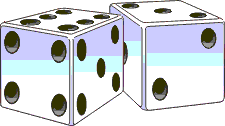planEASe Risk Analysis is based on a recognized technique in the literature of Operations Research known as "Monte Carlo Simulation". The basic concept of the technique is best described through illustration. We all know that a normal six-sided die with the numbers one through six on each of the faces has an equal chance of showing any of the six numbers on any one roll. Statisticians would say that the results of a roll are "uniformly distributed" between one and six, and that the results of any one roll of the die represented a "random number" sampled from that uniform distribution. This is very fancy language for a very simple concept, but the language becomes more useful as we get deeper into the Monte Carlo method.

Now, suppose that you wanted to know what the chances were that the numbers on a normal pair of dice would total seven when rolled. While there are many ways to solve this question mathematically, one simple method would be to roll the dice many times and count the proportion of times that the total is actually seven. If for instance, you rolled them 36 times and they totaled seven on six occasions, you might deduce that the chances of getting a seven were one in six, or 16.7%. As it happens, you would be exactly right in this instance. However, the dice could have shown seven on ten occasions. In this case, the results of your "simulation" would have been misleading, unless you had the judgment to take the results with a grain of salt. The results of rolling a pair of dice are random after all, and the chances of rolling a seven the precise six times required here are rather small.

If, however, we were interested in the average number shown on the dice in the same 36 rolls, you typically would find that the total of the numbers on the dice divided by the 36 rolls was close to seven. In other words, your simulation was a rather good predictor of the average result, while not necessarily giving you accurate information about the probability of any individual result occurring.

To continue the illustration, suppose that you were interested in describing the "spread" of your simulation results. One common method of doing this is to show the results in what is known as a "bar graph" or "histogram". Suppose that the results of your 36 rolls were: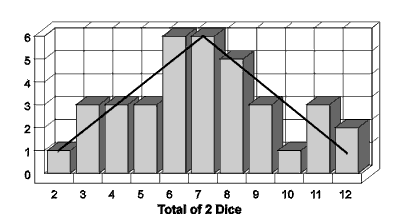• 1 two
3 threes
3 fours
3 fives
6 sixes
6 sevens
5 eights
3 nines
1 ten
3 elevens
2 twelves

The bar graph of these results is shown to the right of the table. Each bar is proportionally as high as the number of times that the result occurred, so that the bar for the result of three is three times as high as the bar for the result of two. Such a graph is a useful tool to describe the results of your simulation, although you would not believe that it accurately represented the chances of rolling a particular total, since the results are random. One thing you might do, however, is draw a smooth line over the results, as has been done here, and think that such a line might come close to the tops of a bar graph of a "perfect" simulation". In this case, you would be right, since the triangle shape is the actual underlying "probability distribution" of the sum of two die.

While the bar graph is a good picture of the results of the simulation, statisticians typically use two particular numbers to describe the same thing in summarized form. The first statistic is the "mean" or average result. This is determined by adding all the results, and dividing the total by the number of trials. In this case, the average result is 290/36 or 8.06. The second statistic is called the "standard deviation". It is a measure of the "spread" of the distribution, and is, mathematically, the square root of the sum of the squares of the deviations from the mean divided by the number of trials. While that is a confusing definition, the use of the statistic in the context of Risk Analysis is quite simple. Since the standard deviation measures the spread of the results, it is a good measure of the amount of risk in the simulation results.

Although the dice illustration is quite simple, a statistician would say that we have just conducted a Monte Carlo Simulation for 36 trials in order to describe the probability distribution of the total shown on a pair of dice. In order to do so, we have sampled two random numbers from a uniform probability distribution between one and six, and performed a mathematical operation (adding the two numbers together) on the pair of random numbers. The Risk Analysis of Analytic Associates is performed in exactly this fashion. However, there are a few differences due to the nature of the real-life situation we are simulating.

The uniform probability distribution for the number on a die is relatively unusual in real life. First, the sum can only assume integer values, whereas most variables are "continuous" values. The inflation rate in the economy, for instance, can be 7.033% or 9.445% ... it is not restricted to integers. Secondly, the shape itself is unusual in that most uncertain variables in real life are distributed as some kind of "bell shaped curve".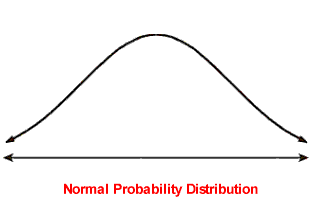There are several bell shaped curves defined in the mathematics of probability. The most commonly known is the "normal" distribution, shown here. It is "symmetric", in that the left side of the curve is a mirror image of the right side. Another characteristic is that the curve never crosses the bottom line, but rather trails away endlessly. In other words, if we were to say that the inflation rate were normally distributed with an average of 7%, we would implicitly be saying that there was a real possibility, however slight, that inflation could be -1,000%.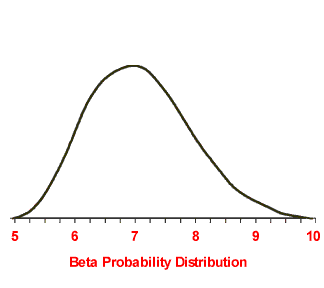In other words, the beta distribution curve has its highest point at the "most likely" amount for the random variable, seven percent, and there is no possibility under this distribution of inflation amounts lower than five percent and higher than ten percent. Note that the distribution is slightly "skewed" because the most likely point is not midway between the outside limits.

planEASe Risk Analyses use beta distributions to describe the user’s uncertain assumptions, just as the illustration used a uniform distribution to describe the number on the die. Thus, when a random number is sampled from the beta distribution, it is more likely to be close to the most likely value than the tails of the distribution. The reason that we use beta distributions is not at all mathematical. Quite simply, we believe that this distribution best describes the shape of what the user really means when he says that inflation will be about 7% and certainly between 5% and 10%.

Monte Carlo Simulation for Risk Analysis is conducted almost exactly as in the dice illustration. First, the random numbers are sampled for each of the uncertain assumptions. This is analogous to rolling the dice. Secondly, the random numbers obtained are used together with the other assumption values to perform the basic analysis. This mathematical operation is analogous to totaling the numbers on the dice in the illustration. The measure requested by the user is then recorded in a table for display in a bar graph, just as we did for the total shown on the dice.

Before discussing Risk Analysis as performed by planEASe, we should define what is meant by the term "risk" itself. Most investors think of the risk in their investments in terms of whether there is a significant chance of losing money. Such an investment is termed "risky". However, in a more general sense, risk relates to the range of possible results of the investment. In this sense, an investment with possible rates of return between 10% and 50% is "riskier" than an investment in a bond with a guaranteed 8% rate of return held to maturity. The purpose of Risk Analysis here is to evaluate the range and probability for the rate of return on the investment, so "risk" is treated here in the more general sense.

As you enter assumptions into a planEASe analysis, there are many whose values are inherently uncertain. For example, look at the TEST assumption set for the RU models shipped with planEASe. Some of the values in this assumption set are shown in the table at the top of the screen below in the "Most Likely" column. For instance, the TEST Assumption Set assumes that the user will hold the Sample Apartments for four years, and then sell the property for five times its Gross Income at that time. However, it would be sheer happenstance if the property were sold for exactly five times the gross income in exactly four years. These assumption values represent educated guesses, not accurate predictions.

In the case of the Sample Apartments, the user has recognized this weakness in the Basic Analysis, and has asked for a Risk Analysis to investigate the risk involved in the Rate of Return Before Tax. He has examined his assumption values and selected those which he considers to be subject to uncertainty. For example, although he thinks that the Gross Income Multiplier assumption value of five times is a good estimate, he believes that the eventual multiple could be anywhere from four times at the lowest, to six and a half times at the highest. The list of all of these risk assumptions selected by the user is shown in the upper portion of the screen. The list shows the lowest, most likely, and highest values that the user believes are possible for the assumptions. Implicitly then, he is also saying that the values for the other assumptions in the analysis are fixed, and will not vary.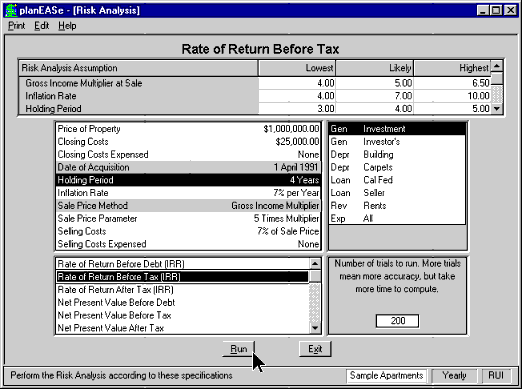While the quantified range of the user’s uncertainty for his assumed values is certainly useful information, it does not answer the question with which he is most concerned ... what does the uncertainty for the assumptions mean in terms of the ultimate rate of return. Obviously, he would like to see all of these assumption values combined in some fashion to see the range of possible rates of return considering those uncertainties. This is where "Monte Carlo Simulation" comes into play. planEASe Risk Analysis uses this technique to project the probability distribution of the rate of return after tax from the assumed values.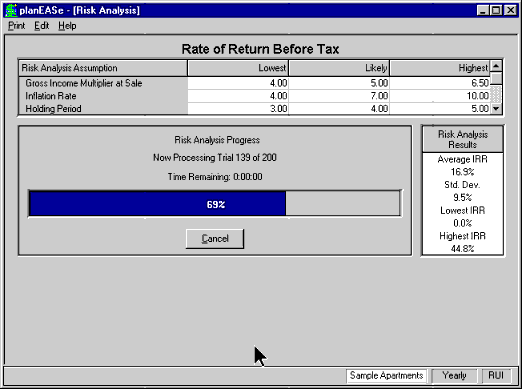The screen below has been obtained after conducting a Monte Carlo Simulation of the Real Estate Investment Analysis for two hundred trials (in progress in the screen above). For each of these trials, the risk analysis process selects a random number from the beta probability distribution for each of the uncertain assumptions. The selection of these random numbers is such that each number can assume any value within the lowest to highest range for that assumption, but more likely will be around the most likely value. Thus a bar graph of the one hundred random numbers selected for any one assumption would look like the corresponding beta distribution, subject to the randomness of the process.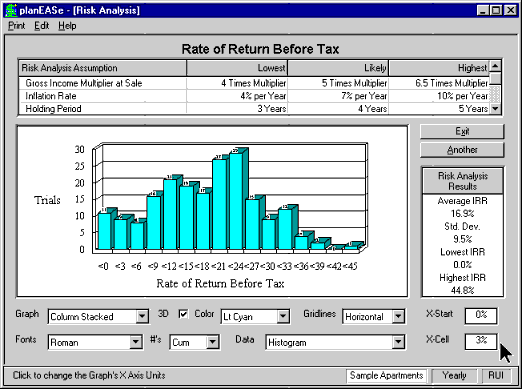When the random numbers for the uncertain assumptions have been selected for one of the trials, the basic analysis is completed using those assumption values. In this case, the user has requested that the Risk Analysis be performed for the Rate of Return Before Tax (just as with Sensitivity Analysis, Risk Analysis may be conducted for any of the measures in the model). Accordingly, that rate of return is recorded in a table after each of the hundred trials. A bar graph of the rates of return obtained in the one hundred trials is shown in this screen. It shows, for example, that there were ten rates of return below zero, eight between 0% and 3%, and seven more between 3% and 6%.

Some useful statistics for the hundred trials on the right side of the screen. The average rate of return was 16.9% as opposed to the 15.4% for the same measure in the Basic Analysis. Some of this variation can be ascribed to the randomness of the simulation. Additionally, some of the distributions for the uncertain assumptions are skewed, or asymmetrical. It is a characteristic of such distributions that the means of the distributions are different from the most likely or highest points. Mathematically, this means that the average of our hundred trials should be different than the 15.4%.

The last two statistics shown are the lowest and highest rates of return obtained in the hundred trials: 0.0% and 44.8% in this case. While these are useful numbers, they should be interpreted with extreme care. For instance, if we had conducted the simulation a thousand times, we almost surely would have obtained rates of return higher than 44.8% In other words, these two numbers do not show the lowest and highest rates of return possible under the assumptions. Those lowest and highest rates could only be obtained by requesting basic analyses using only the most pessimistic and most optimistic assumption values from the list at the top of the page. Even then, those rates of return would represent the possible range of rates of return only if the assumption ranges were all correct in actual fact, which is extremely unlikely. In short, the Risk Analysis is not intended to show the entire range of possible investment results, but rather is meant to give you an approximate picture of the probability of those results.

The lowest rate of return obtained in this simulation was 0.0%. planEASe does not compute a rate of return if the sum of the cash flow involved is negative, but rather records zero percent for that case. In this case, six of the hundred trials resulted in the investor not recovering his invested funds. Users interested in how much money was lost by the investor in such cases may request a Risk Analysis for the Net Present Value of the same cash flow using a zero discount rate.

Some useful conclusions may be drawn from the bar graph itself. For instance, there are 25 rates of return less than 6%. One could say, then, that there is about a 12% chance of making less than 6% on the investment. Similarly, there is an even chance of obtaining a rate of return greater than 17%, and a 5% possibility of losing money on the investment. Considering the wide ranges chosen for the assumption values, this analysis should provide considerable comfort for the user who is worried about the possible "downside" risk in the investment.

There are some limitations in Monte Carlo Risk Analysis which should be of concern to you. For instance, Monte Carlo Simulation uses random numbers for the risk assumption values. This causes the results of the simulation to be slightly unreliable. This unreliability becomes smaller and smaller as the number of trials is increased. Experience with the technique indicates that one hundred trials gives a good prediction for the mean and standard deviation of the resulting probability distribution, but does not, typically, show the distribution shape or the length of the tails accurately. Two hundred trials typically results in a smoother distribution, and five hundred trials typically give an extremely smooth distribution with good definition of the tails.

Another limitation of the process is that the simulation assumes that all the assumption values and ranges are accurate, and also assumes that the assumptions are independent of one another. Accuracy in the all the assumption values is obviously impossible. Independence of the assumption values is also typically questionable. For instance, on any single trial, the simulation could choose a 4% Inflation Rate and a 6.5 sale multiple to go with it. Clearly, the chances of selling the property for 6.5 times gross when inflation has been low would be extremely unlikely.

While Risk Analysis has some significant limitations due to the technique involved, it is an extremely useful tool for investigating the amount of risk involved in an investment. By quantifying the variability of the results of the investment, it allows you to properly portray the real nature of the investment. It is a truism that real estate investments are "risky", but Risk Analysis allows you to quantitatively measure that risk.

### Perform Monte Carlo Risk Analysis with any assumptions you choose versus any measure, such as Rate of Return (IRR or MIRR), Net Present Value (NPV), etc. Risk Analysis allows you to investigate how these measures vary with a change in assumptions like Holding Period, Cap Rate at Sale, Renewal Probability, Vacancy, TI's, etc. Risk Analysis provides a one page table and graph which shows the probability of achieving any level for the chosen measure.